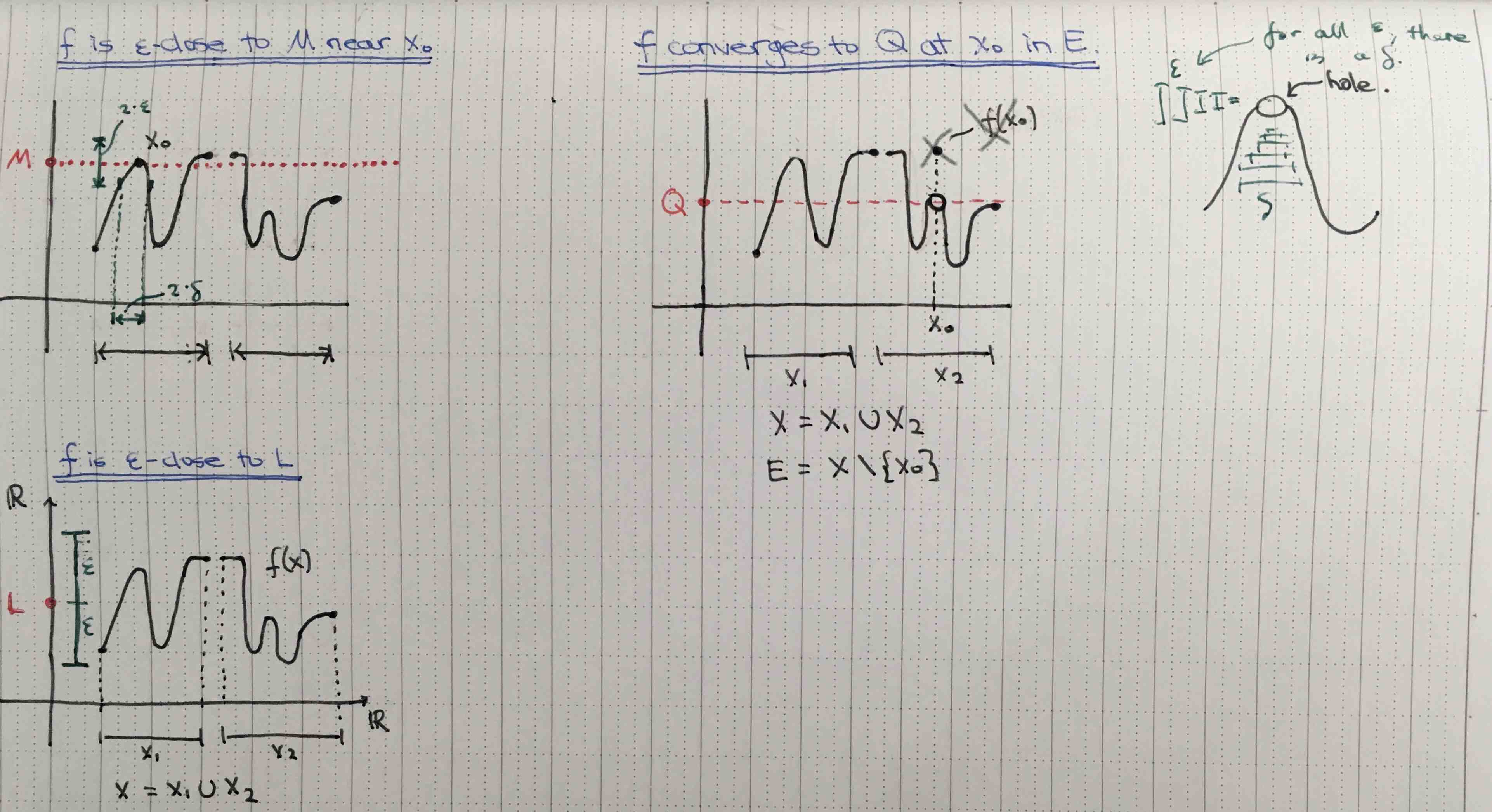Show Question
Math and science::Analysis::Tao::09. Continuous functions on R

# Function convergence at a point, definition

#### ε-closeness, of a function to a real

Let $$X$$ be a subset of $$\mathbb{R}$$, let $$f : X \to \mathbb{R}$$ be a function, let $$L$$ be a real, and let $$\varepsilon > 0$$ be a real.

We say that the function $$f$$ is ε-close to $$L$$ iff $$f(x)$$ is ε-close to $$L$$ for every $$x \in X$$.

#### Local ε-closeness, of a function to a real

Let $$X$$ be a subset of $$\mathbb{R}$$, let $$f : X \to \mathbb{R}$$ be a function, let $$x_0$$ be an adherent point of $$X$$, let $$L$$ be a real, and let $$\varepsilon > 0$$ be a real.

We say that $$f$$ is ε-close to $$L$$ near $$x_0$$ iff there exists a $$\delta > 0$$ such that $$f$$ becomes ε-close to $$L$$ when restricted to the set $$\{x \in X : |x - x_0| < \delta \}$$.

### Convergence of a function at a point

Let $$X$$ be a subset of $$\mathbb{R}$$, let $$f : X \to \mathbb{R}$$ be a function, let $$E$$ be a subset of $$X$$, let $$x_0$$ be an adherent point of $$E$$, let $$L$$ be a real, and let $$\varepsilon > 0$$ be a real.

We say that $$f$$ converges to $$L$$ at $$x_0$$ in $$E$$, and write $$\lim_{x \rightarrow x_0; x \in E} f(x) = L$$, iff $$f$$, after restricting to $$E$$, is ε-close to $$L$$ near $$x_0$$ for every $$\varepsilon > 0$$. If $$f$$ does not converge to any number $$L$$ at $$x_0$$, we say that $$f$$ diverges at $$x_0$$, and leave $$\lim_{x \rightarrow x_0; x \in E} f(x)$$ undefined.

These definitions are separated into 2 paragraphs due to the many supporting objects required.

#### Laxness of the phrase "restricting the function $$f$$ to the range $$X$$"

Tao talks on page 219 about the laxness of this phrase. What is mean is that we are referring to another distinct function $$f|_X : X \to B$$ defined by $$f|_X(x) = f(x)$$.

#### The familiar structure of closeness

We are getting used to the proceedure now: define a notion of ε-closeness, a notion of restricted ε-closeness, and finally, a notion of having restricted ε-closeness for all ε.Correction to image: either $$x_0 \notin X$$ or $$f(x_0) = Q$$. If $$f(x_0)$$ is something other than $$Q$$, then $$f$$ wouldn't be locally close to $$Q$$ near $$x_0$$ for any $$\varepsilon > |Q - f(x_0)|$$.

### Example

Let $$f : [1, 3] \to \mathbb{R}$$ be the function $$f(x) = x^2$$.

• This function is 5-close to 4.
• It is not 0.1 close to 4.
• it is 0.1 close to 4 near 2 (when $$f$$ is restricted to the set $$\{ x \in [1, 3] : |x - 2| < 0.01 \}$$ it is 0.1 close to 4).
• It is 0.1 close to 9 near 3. (Notice the half-open interval being used.)
• It converges to 9 at 3 in $$[1,3]$$ (when not restricted at all).

p221-222